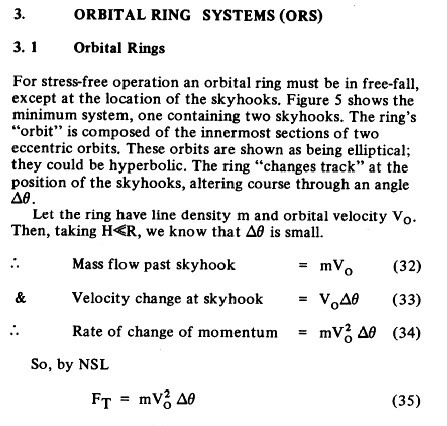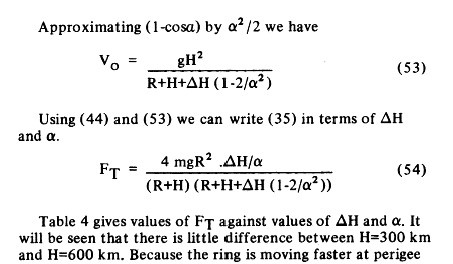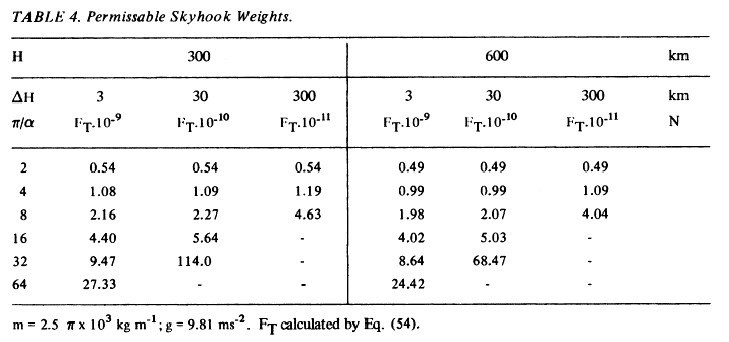# Questions about orbital ring math from Birch 1982 paper

I have been reading the original Paul Birch paper published in JBIS 1982. I have been using this copy found here.

In particular I am wondering about generalizing the ideas, like for a different planet or body what would the forces look like? In short, I wondered what a chandelier city could look like in a hypothetical scenario.

In section 3 of the paper Birch presents an equation and then breaks the terms into constituents which are then brought back together on the next page.$$F_T$$ is defined elsewhere and is the force applied at a tether 'anchor' I suppose, a location where the ring is directed into another orbit and the momentum transferred to holding a tether aloft. Measured in newtons.

$$\Delta \Theta$$ is the angle change at one of the anchor stations

This is the base equation. Over the next two pages he develops the terms and then is able to rewrite $$F_T$$ in terms of $$\Delta H$$, the height difference in the perigee of the new orbit and $$\alpha$$, the angle of the orbit of the ring after exiting a skyhook station. I have attached a screen shot of this final equation.He provides 'Table 4-Permissable Skyhook Weights' with a set of values and the resultant $$F_T$$ weight of a skyhook tether in N (newtons).I then have been setting up my work thusly and having some trouble with the equation:

$$m$$ = given in table footnote as $$2.5 \pi * 10^3$$

$$g$$ = acceleration due to gravity $$9.8 m/s^2$$

$$R$$ = radius of earth at equator of $$6378$$ km

$$\Delta H$$ = as given in the table is $$\frac{\pi}{\alpha}$$ and provided as $$2$$ for the first example.

$$F_T = \frac{4mgR^2 * \frac{\Delta H}{\alpha}}{(R+H)(R+H+\Delta H*(1-\frac{2}{\alpha ^2})}$$

I am having trouble replicating the data in the table. Using the values there I have interpreted $$\frac{\Delta H}{\alpha}$$ to be $$\frac{3}{2}$$ and when that did not produce the expected result I tried to use $$\frac{\pi}{\alpha}$$. I am not sure if this is the error in the calculation.

From the table I am expecting a result for $$H=300$$, $$\Delta H=3$$, $$\frac{\pi}{\alpha}$$ of $$540000000 * 10^{-9}$$ or $$0.54$$. The result I am getting is substantially different.

Is anyone familiar with the math behind the concept to offer a hand to help me understand where I've gone wrong?

• I love the cover art (first page of PDF)
– uhoh
Jan 25, 2022 at 4:32
• Please edit your question and supply the actual substantially different value you are getting so that someone can help. Jan 25, 2022 at 12:50
• @OrganicMarble Thank you. I should've made things more clear in my question that I wasn't even sure I had the right values as inputs. From looking at the response I see that I was incorrect on two values. Jan 25, 2022 at 22:36

The table should be understood as follows: the first data column (0.54, 1.08, ...) contains the values of $$F_T\cdot 10^{-9}$$ in Newtons for different values of $$\pi/\alpha$$ (2, 4, ...) in the case where $$\Delta H$$ is 3 km; the second column (0.54, 1.09, ...), for the case where $$\Delta H$$ is 30 km, etc.
For example, if we take $$H = 300~\text{km}$$, $$\Delta H = 3~\text {km}$$ and $$\alpha = \pi/2$$, then $$F_T = \frac{4~\cdot~ 2.5\pi\cdot10^3~\text{kg m}^{-1}~\cdot~9.8~\text{m s}^{-2}~\cdot~ (6371000~\text{m})^2~\cdot~ \frac{3000~\text{m}}{\pi/2}}{6821000~\text{m}~\cdot~(6821000~\text{m} ~+~3000~\text{m}~\cdot~(1 - \frac{8}{\pi^2}))} \approx 5.4\cdot 10^8~\text{N},$$ or $$F_T\cdot 10^{-9}$$ is $$0.54~\text{N}$$.
• This is a perfect explanation. I see that I was incorrect in my understanding of the $\frac{\pi}{\alpha}$ terms in the equation. Jan 25, 2022 at 22:50
• Would I be correct in thinking that the $\frac{\pi}{\alpha}$ term is the number of radians between the previous orbit modified by the 'station' and the perigee of this new orbit? Jan 25, 2022 at 22:58
• @b00klegger If I understand Fig. 6 correctly, $\alpha$ is the angle (in radians) between a station and an adjacent orbit's perigee, when seen from the Earth's center. If perigees are located at midpoints between two stations, then $\frac{\pi}{\alpha}$ is the total number of stations along the Earth's circumference. Jan 26, 2022 at 8:47